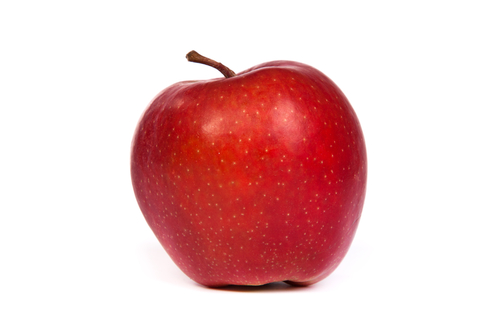#Teacher Note: Print() is Your Friend¶

The goal of this stage of learning about programming is to develop a mental model of how the program works. Can students look at a program and predict what’s going to happen? Can they figure out the values of the variables? Feel free to insert lots of `print()` function calls. Have students make a prediction about variable values, then insert `print()` calls to display the variable values, and run the program to find out whether the prediction is right. Run this version to see what gets printed.

The following is the correct code for printing the value of number and the sum each time through the loop, but it is mixed up. The code should initialize the accumulator, create the list of numbers, and then loop through the list of numbers. Each time through the loop it should print the value of number, add the value of number to the accumulator, and then print the current sum. Drag the blocks from the left and put them in the correct order on the right. Don’t forget to indent blocks in the body of the loop. Just drag the block further right to indent. Click the <i>Check Me</i> button to check your solution.</p>

```        sum = 0
---
numbers = range(0,101,2)
---
for number in numbers:
---
print("Number:",number)
---
sum = sum + number
---
print(sum)
```
You have attempted of activities on this page